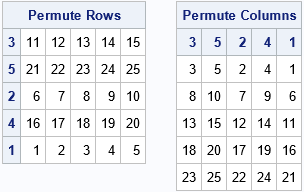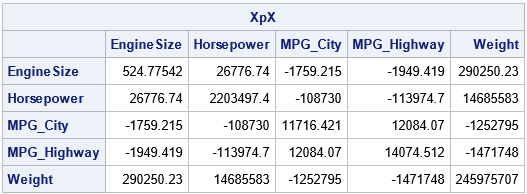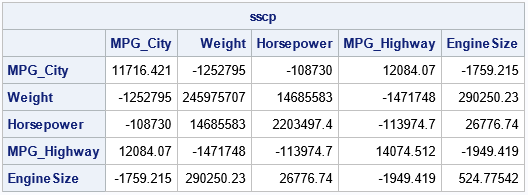Do you ever use a permutation matrix to change the order of rows or columns in a matrix? Did you know that there is a more efficient way in matrix-oriented languages such as SAS/IML, MATLAB, and R? Remember the following tip:
Never multiply with a large permutation matrix! Instead, use subscripts to permute rows and columns.

This article explains why it is not necessary to multiply by a permutation matrix in a matrix language. The advice is similar to the tip in the article, "Never multiply with a large diagonal matrix," which discusses an efficient way to use diagonal matrices in matrix computations. That article recommends that you never multiply with a large diagonal matrix. Instead, use elementwise multiplication of rows and columns.

### Permutation matrices

Permutation matrices have many uses, but their primary use in statistics is to rearrange the order of rows or columns in a matrix. A previous article shows how to create a permutation matrix and how to use it to permute the order of rows and columns in a matrix. I ended the article by noting that "there is an easy alternative way" to permute rows and columns: use subscript notation. Subscripts enable you to permute rows and columns efficiently and intuitively. If you use matrix multiplication to permute columns of a matrix, you might not remember whether to multiply the permutation matrix on the left or on the right, but if you use subscripts, it is easy to remember the syntax.

The following example defines a 5 x 5 matrix, A, with integer entries and a function that creates a permutation matrix. (The permutation matrix is the transpose of the matrix that I used in the previous article. I think this definition is easier to use.) If you multiply A on the right (A*Q), you permute the columns. If you multiply A on the left (Q`*A), you permute the rows, as shown:

```proc iml; A = shape(1:25, 5, 5); /*{ 1 2 3 4 5, 6 7 8 9 10, ... 21 22 23 24 25} */ v = {3 5 2 4 1}; /* new order for the columns or rows */   /* Method 1: Explicitly form the permutation matrix */ /* the following matrix permutes the rows when P` is used on the left (P`*A) Use P on the right to permute columns. */ start PermutionMatrixT(v); return( I(ncol(v))[ ,v] ); finish; Q = PermutionMatrixT(v); C = A*Q; /* permute the columns */ R = Q`*A; /* permute the rows */ ods layout gridded advance=table columns=2; print R[r=v L="Permute Rows"], C[c=v L="Permute Columns"]; ods layout end;```This method explicitly forms a permutation matrix to permute the rows and/or columns. To permute the rows of an N x p matrix, the permutation matrix is an N x N matrix, and the matrix multiplication requires O(N2p) floating-point operations. To permute the columns, the permutation matrix is p x p, and the matrix multiplication requires O(Np2) operations.

### Permutation of indices for subscripts

In contrast, if you permute a matrix by using subscript indices, no floating-point operations are needed. The syntax A[v,] permutes the rows of the matrix A by using the indices in the vector v. The syntax A[,v] permutes the columns of A. The syntax A[v,v] permutes both the rows and the columns. Here is the previous permutation, but without using permutation matrices:

```/* Method 2: Specify subscript indices to form the permutation matrix */ C = A[ ,v]; /* permute the columns */ R = A[v, ]; /* permute the rows */ print R[r=v L="Permute Rows"], C[c=v L="Permute Columns"];```

The output is the same and is not shown. No computations are required, only copying data from one matrix to another. For v = {3 5 2 4 1}, the first syntax means, "copy the columns in the order, 3, 5, 2, 4, and 1." The second syntax specifies the order of the rows. You don't have to remember which side of A to put the permutation matrix, nor whether to use a transpose operation.

### An application: Order of effects in a regression model

In my previous article, I used a correlation matrix to demonstrate why it is useful to know how to permute rows and columns of a matrix. Another application is the order of columns in a design matrix for linear regression models. Suppose you read in a design matrix where the columns of the matrix are in a specified order. For some statistics (for example, the Type I sequential sum of squares), the order of the variables in the model are important. The order of variables is also used in regression techniques such as variable selection methods. Fortunately, if you have computed the sum of squares and crossproducts matrix (SSCP) for the variables in the original order, it is trivial to permute the matrix to accommodate any other ordering of the variables. If you have computed the SSCP matrix in one order, you can obtain it for any order without recomputing it.

The SSCP matrix is part of the "normal equations" that are used to obtain least-squares estimates for regression parameters. Let's look at an example. Suppose you compute the SSCP matrix for a data matrix that has five effects, as shown below:

```/* permute columns or rows of a SSCP matrix (XpX) */ use sashelp.cars; varNames = {'EngineSize' 'Horsepower' 'MPG_City' 'MPG_Highway' 'Weight'}; read all var varNames into Z; close;   X = Z - mean(Z); /* center the columns */ XpX = X`*X; print XpX[r=varNames c=varNames];```Now, suppose you want the SSCP matrix for the same variables in a different order. For example, you want the order {'MPG_City' 'Weight' 'Horsepower' 'MPG_Highway' 'EngineSize'}; which is the permutation {3, 5, 2, 4, 1} of the original ordering. You have two choices: permute the columns of the design matrix and recompute the SSCP or permute the existing SSCP matrix. The first way is intuitive but less efficient. It is shown below:

```/* inefficient way: create new design matrix, Y, which permutes columns of X. Then compute SSCP. */ v = {3 5 2 4 1}; Q = PermutionMatrixT(v); Y = X*Q; sscp = Y`*Y; /* = Q`*X`*X*Q = Q`*(X`*X)*Q */ pVarNames = varNames[,v]; /* get new order for var names */ print sscp[r=pVarNames c=pVarNames];```The second way is a one-liner and does not require any computations. You don't have to form the permutation matrix, Q. You only need to use subscripts to permute the new SSCP:

```/* efficient way: don't form Q; just permute the indices */ sscp2 = XpX[v,v]; print sscp2[r=pVarNames c=pVarNames];```

The output is exactly the same and is not shown. After you have obtained the SSCP matrix in the new order, you can use it to compute regression statistics. If you are solving the normal equations, you also need to permute the right-hand side of the equations, which will be equal to Q`*X`*y.

### Summary

In summary, you rarely need to explicitly form a permutation matrix and use matrix multiplication to permute the rows or columns of a matrix. In most situations, you can use subscript indices to permute row, columns, or both rows and columns.

Share